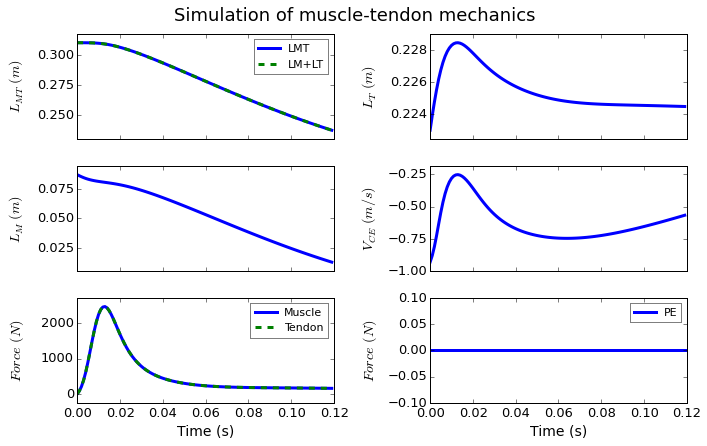# Musculoskeletal modeling and simulation¶

Marcos Duarte, Renato Watanabe
Laboratory of Biomechanics and Motor Control (http://demotu.org/)
Federal University of ABC, Brazil

We will now simulate the dynamics of the musculoskeletal system with muscle dynamics. You should have read Muscle modeling and Muscle simulation. In addition, chapter 4 of Nigg and Herzog (2006) is a good introduction to this topic.

Let's start with the simplest case, a one-link rigid body with one degree of freedom and one muscle.

Let's simulate the extension movement of the knee. This problem is based on an example from chapter 4 of Nigg and Herzog (2006). We will model the task as a planar movement, the leg and foot as one rigid segment and the rest of the body fixed, the knee as a revolute joint with fixed axis of rotation and one degree of freedom, and only one muscle as knee extensor, as illustrated in the figure below.Figure. Planar model of the knee with one degree of freedom, one extensor muscle, and the corresponding free-body diagram for the leg. $\theta$: knee angle with horizontal; $F_M$ force of the quadriceps muscle; $F_J$: force at the knee joint; $r_M$: moment arm of the quadriceps; $r_{cm}$: distance to the knee joint of the center of mass of the leg+foot segment; $mg$: weight of the leg+foot; $L_{MT0}$: muscle-tendon unit length at $\theta=90^o$.
In :
import numpy as np
%matplotlib inline
import matplotlib
import matplotlib.pyplot as plt
matplotlib.rcParams['lines.linewidth'] = 3
matplotlib.rcParams['font.size'] = 13
matplotlib.rcParams['lines.markersize'] = 5
matplotlib.rc('axes', grid=False, labelsize=14, titlesize=16, ymargin=0.05)
matplotlib.rc('legend', numpoints=1, fontsize=11)
from scipy.integrate import odeint, ode
# import the muscles.py module
import sys
sys.path.insert(1, r'./../functions')
import muscles


Let's define a function with the system of first-order ODEs:

In :
def onelink_eq(lmthetaomega, t):

# parameters from Nigg and Herzog (2006)
lmt0 = 0.310
rm   = 0.033
rcm  = 0.264
m    = 10
g    = 9.8
I    = 0.1832

lm      = lmthetaomega  # muscle length
theta   = lmthetaomega  # knee position
omega   = lmthetaomega  # knee velocity
lmt     = lmt0 - rm_fun(theta)*(theta - np.pi/2)
lmd     = fv_1_eq(t, lm, lmt)
thetadd = (rm_fun(theta)*fm_eq(lm, lmd, lmt) + rcm*np.cos(theta)*m*g)/I  # acceleration


In :
# define a function for the moment arm
def rm_fun(theta):
"""Vastus intermedius moment arm."""

rm = 0.033

return rm


Functions to calculate the force-velocity relationship:

In :
def fv_1_eq(t, lm, lmt):

lmopt = ms.P['lmopt']
ltslack = ms.P['ltslack']
a = 1
alpha = 0
if lm < 0.1*lmopt:
lm = 0.1*lmopt

lt    = lmt - lm*np.cos(alpha)
fse   = ms.force_se(lt=lt, ltslack=ltslack)
fpe   = ms.force_pe(lm=lm/lmopt)
fl    = ms.force_l(lm=lm/lmopt)
fce_t = fse/np.cos(alpha) - fpe
vm    = ms.velo_fm(fm=fce_t, a=a, fl=fl)

return vm

def fm_eq(lm, lmd, lmt):

fm0  = ms.P['fm0']
lmopt = ms.P['lmopt']
ltslack = ms.P['ltslack']
a = 1
alpha = 0

lt = lmt - lm*np.cos(alpha)
fl = ms.force_l(lm=lm/lmopt)
fpe = ms.force_pe(lm=lm/lmopt)
fm = ms.force_vm(vm=lmd, fl=fl, lmopt=lmopt, a=a) + fpe
fm = fm*fm0

return fm


Let's instantiate the muscles class:

In :
ms = muscles.Thelen2003()
ms.set_parameters()
ms.set_states()
ms.muscle_plot();

The parameters were successfully loaded and are stored in the variable P.
The states were successfully loaded and are stored in the variable S.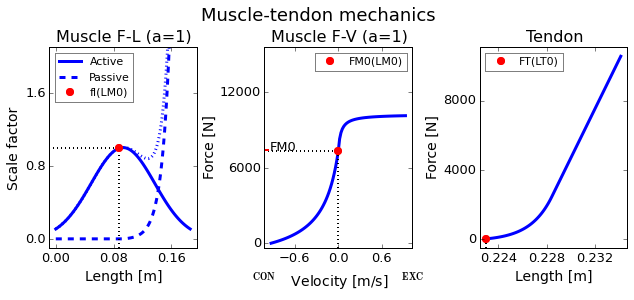Let's input the initial states and use scipy.odeint to solve the ODEs:

In :
lmthetaomega = [0.087, np.pi/2, 0]
time = np.arange(0, .12, 0.001)


Plots of the simulated angular position and velocity:

In :
def sim_plot(time, data, ms):

datam = []
for i, t in enumerate(time):
fm0  = ms.P['fm0']
lmopt = ms.P['lmopt']
ltslack = ms.P['ltslack']
a = 1
alpha = 0
lmt0 = 0.310

lm = data[i, 0]
theta = data[i, 1]
lmt = lmt0 - rm_fun(theta)*(theta - np.pi/2)
lt = lmt - lm*np.cos(alpha)
fl = ms.force_l(lm=lm/lmopt)
fpe = ms.force_pe(lm=lm/lmopt)
fse = ms.force_se(lt=lt, ltslack=ltslack)
fce_t = fse/np.cos(alpha) - fpe
vm = ms.velo_fm(fm=fce_t, a=a, fl=fl, lmopt=lmopt)
fm = ms.force_vm(vm=vm, fl=fl, lmopt=lmopt, a=a) + fpe
d = [t, lmt, lm, lt, vm, fm*fm0, fse*fm0, a*fl*fm0, fpe*fm0, alpha]
datam.append(d)

datam = np.array(datam)

fig, axs = plt.subplots(nrows=1, ncols=2, sharex = True, figsize=(10, 3))
axs.plot(time, data[:, 1]*180/np.pi)
axs.set_xlabel('Time (s)')
axs.set_ylabel('Knee angle $(^o)$')
axs.plot(time, data[:, 2]*180/np.pi)
axs.set_xlabel('Time (s)')
axs.set_ylabel('Knee velocity $(^o/s)$')
plt.tight_layout()
plt.show()

return axs, datam

axs, data2 = sim_plot(time, data, ms)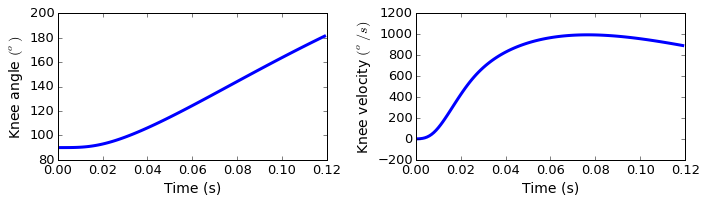And the plots for the simulated muscle mechanics:

In :
axs_lm = ms.lm_plot(data2)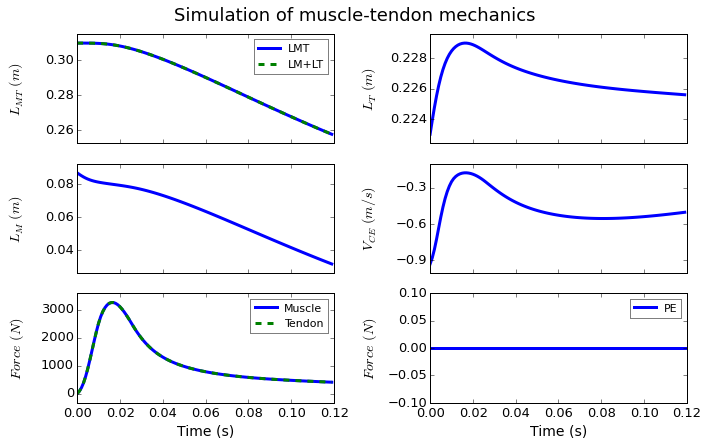### Moment arm is not constant¶

In fact, knee moment arms of the knee extensors are not constant and change with the knee angle. We can import these data from OpenSim. Here is the data for the vastus intermedius moment arm:

In :
vas_int_r = np.loadtxt('./../data/vas_int_r.mot', skiprows=7)
print('Shape of vas_int_r:', vas_int_r.shape)

Shape of vas_int_r: (100, 3)


We need to be able to calculate the moment arm for any angle, not only for the 100 values in these data. Let's fit a spline to these data, which will return a function we can use to calculate the moment arm at any angle:

In :
from scipy import interpolate
S = interpolate.InterpolatedUnivariateSpline(vas_int_r[:, 1], vas_int_r[:, 2])

In :
fig, ax = plt.subplots(nrows=1, ncols=1, figsize=(6, 3))
ax.plot(vas_int_r[:, 1], vas_int_r[:, 2], label='Raw data')
ax.plot(vas_int_r[:, 1], S(vas_int_r[:, 1]), 'r--', label='Spline')
ax.set_xlabel('Knee angle $(^o)$')
ax.set_ylabel('Moment arm (m)')
plt.legend(loc='best')
plt.tight_layout()
plt.show()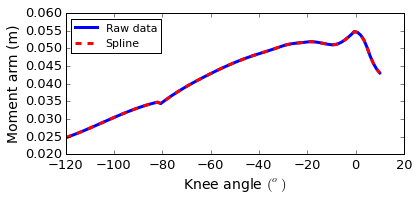It works as expected, we just have to handle the fact that the angle range is different.
Let's define a new function for the moment arm for our simulation:

In :
# define a function for the moment arm
def rm_fun(theta, data=S):
"""Vastus intermedius moment arm."""

rm = S(theta*np.pi/180 - np.pi)

return rm

In :
data = odeint(onelink_eq, lmthetaomega, time)

In :
axs, data2 = sim_plot(time, data, ms)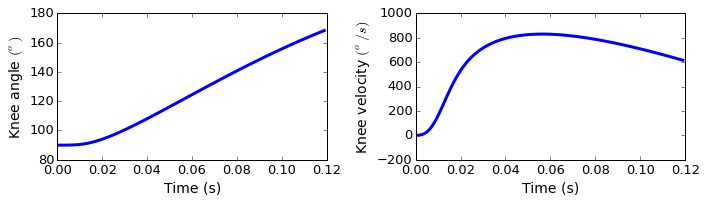In :
axs_lm2 = ms.lm_plot(data2)  # axs_lm